## What are they and what can they do for me?

ScotlandJS
Jaume Sanchez | @thespite

"A computer program that is used to do shading"

"Depicting depth perception
in 3D models or illustrations
by varying levels of darkness"

"A computer program that tells the computer
how to draw something"

Pixar, RenderMan, 1988

Graphic procedures like:
surfacing and texturing,
texture and geometry generation

 Renderman shader: red mesh ``````surface basic() { Ci = (1.0, 0.0, 0.0); Oi = 1; }`````` Renderman shader: red shaded mesh ``````surface simple(color myOpacity = 1;) { color myColor = (1.0, 0.0, 0.0); normal Nn = normalize(N); Ci = myColor * myOpacity * diffuse(Nn); Oi = myOpacity; }``````

Geometry transformation
make things look "3D",
distort objects

Color output
surfaces, textures,
postprocessing

# 3D programming 101

We have an array of values that represent geometry

[ -1, -1, 0 ], [ 1, -1, 0 ], [ 1, 1, 0 ], [ -1, 1, 0 ]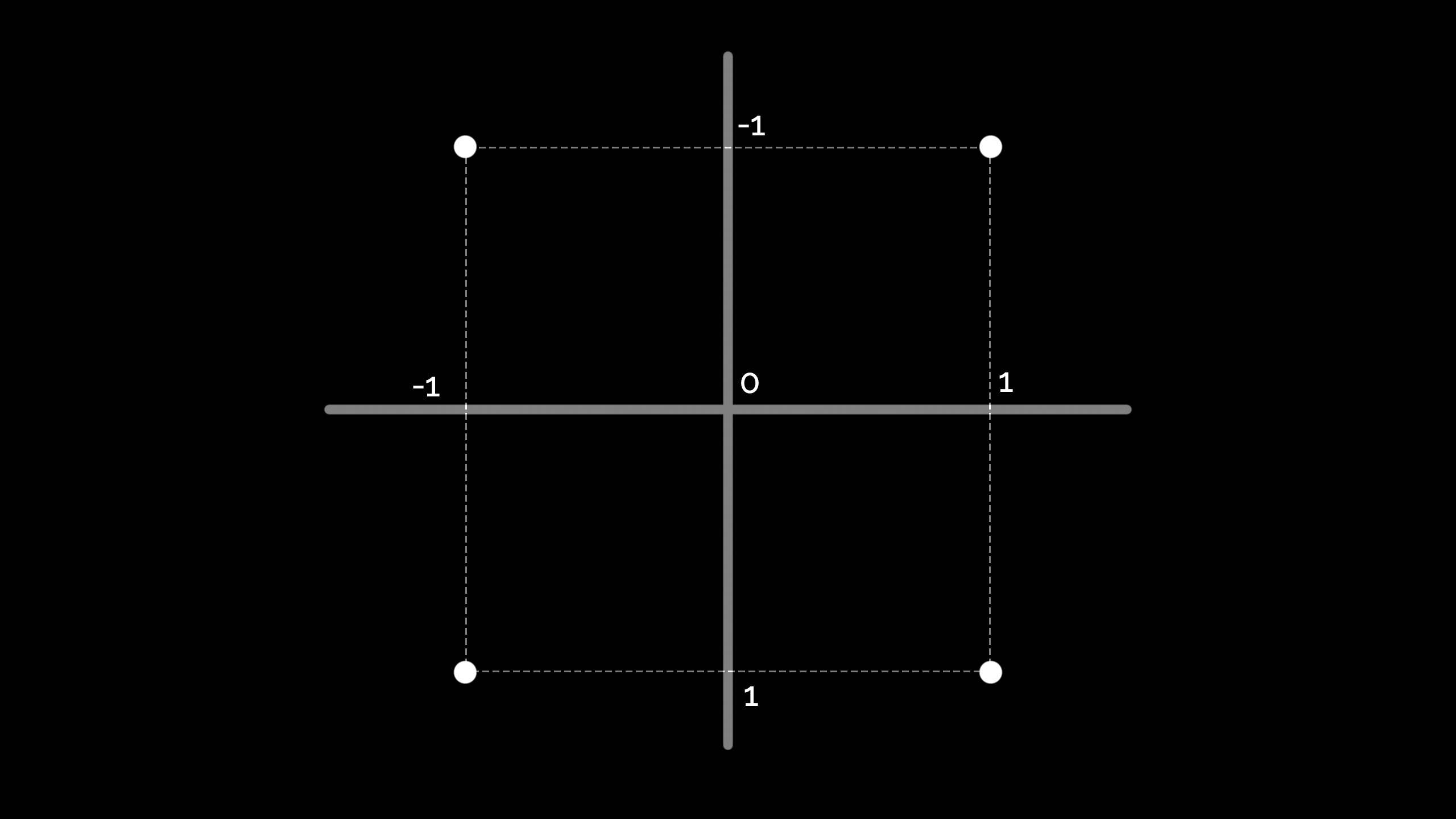In this case, it's the beginning of a square

The vertex shader takes this data, and some other values
(model-view and projection matrix, and others)
and turns the 4 points into 4 projected points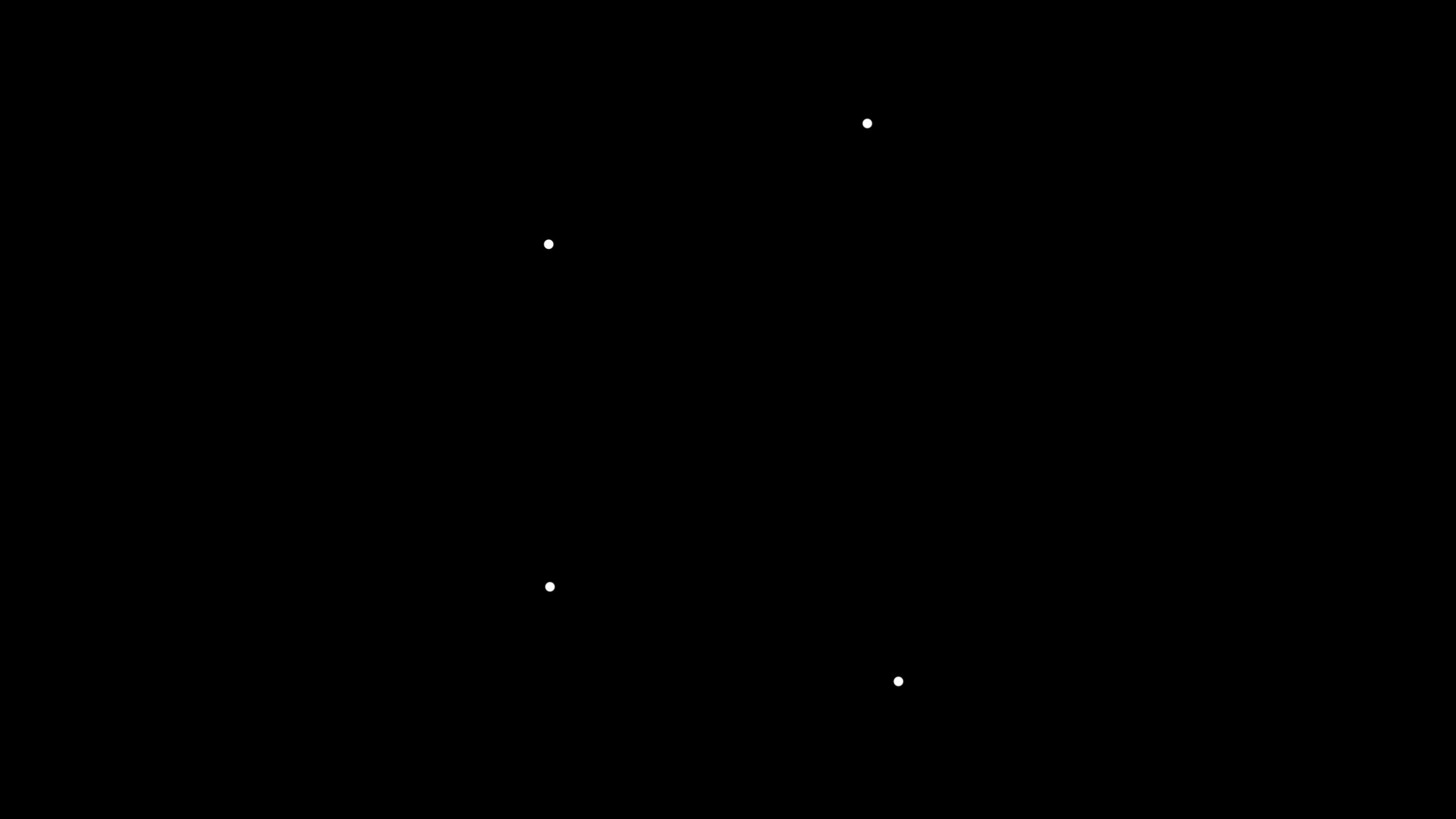These four points exist in mathematical space,
they just represent a concept.

We need to specify how to draw it:
points, wireframe or solid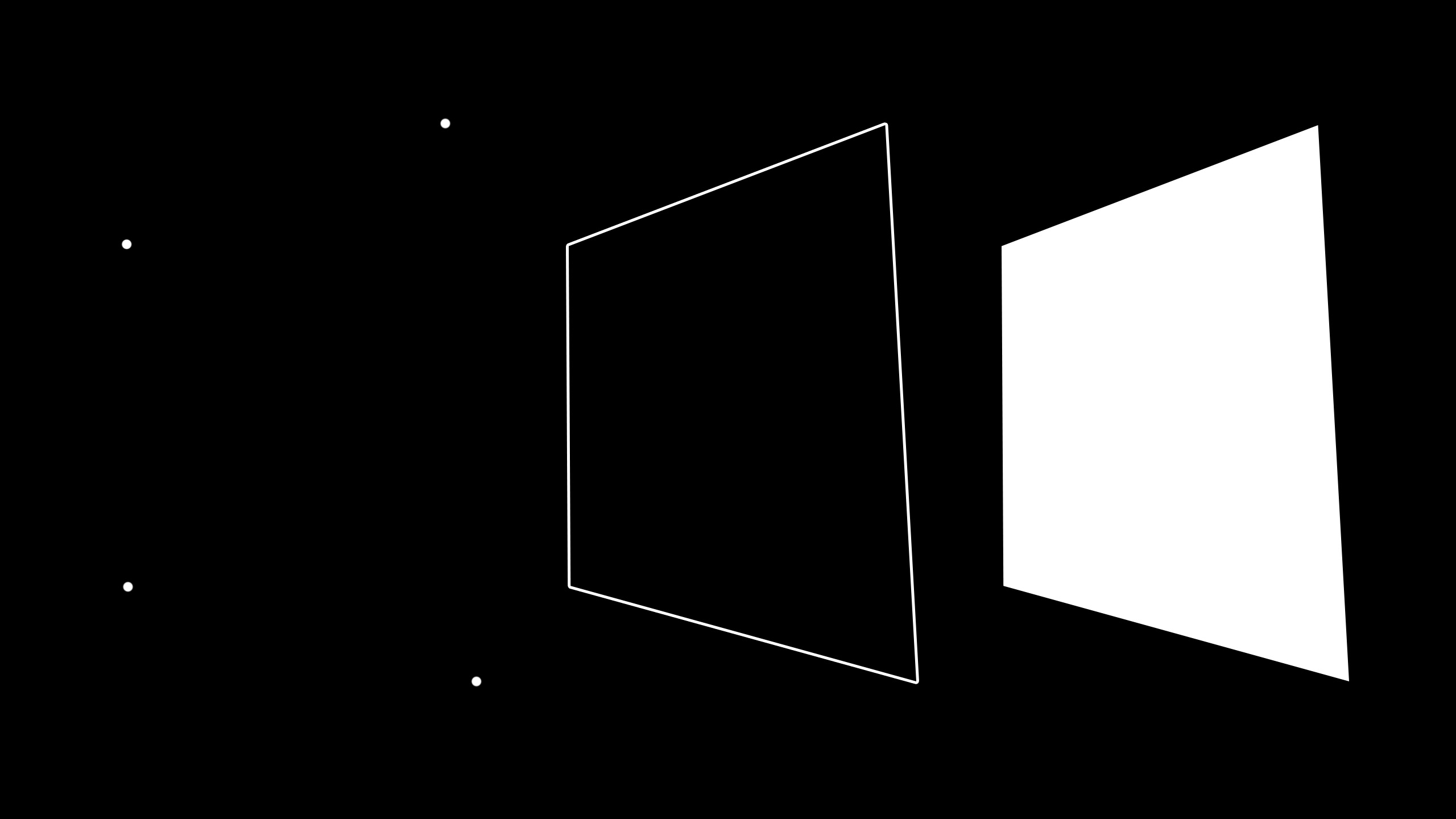We'll choose solid

The solid projected square gets "discretised"
base on the output display,
like your laptop or phone screen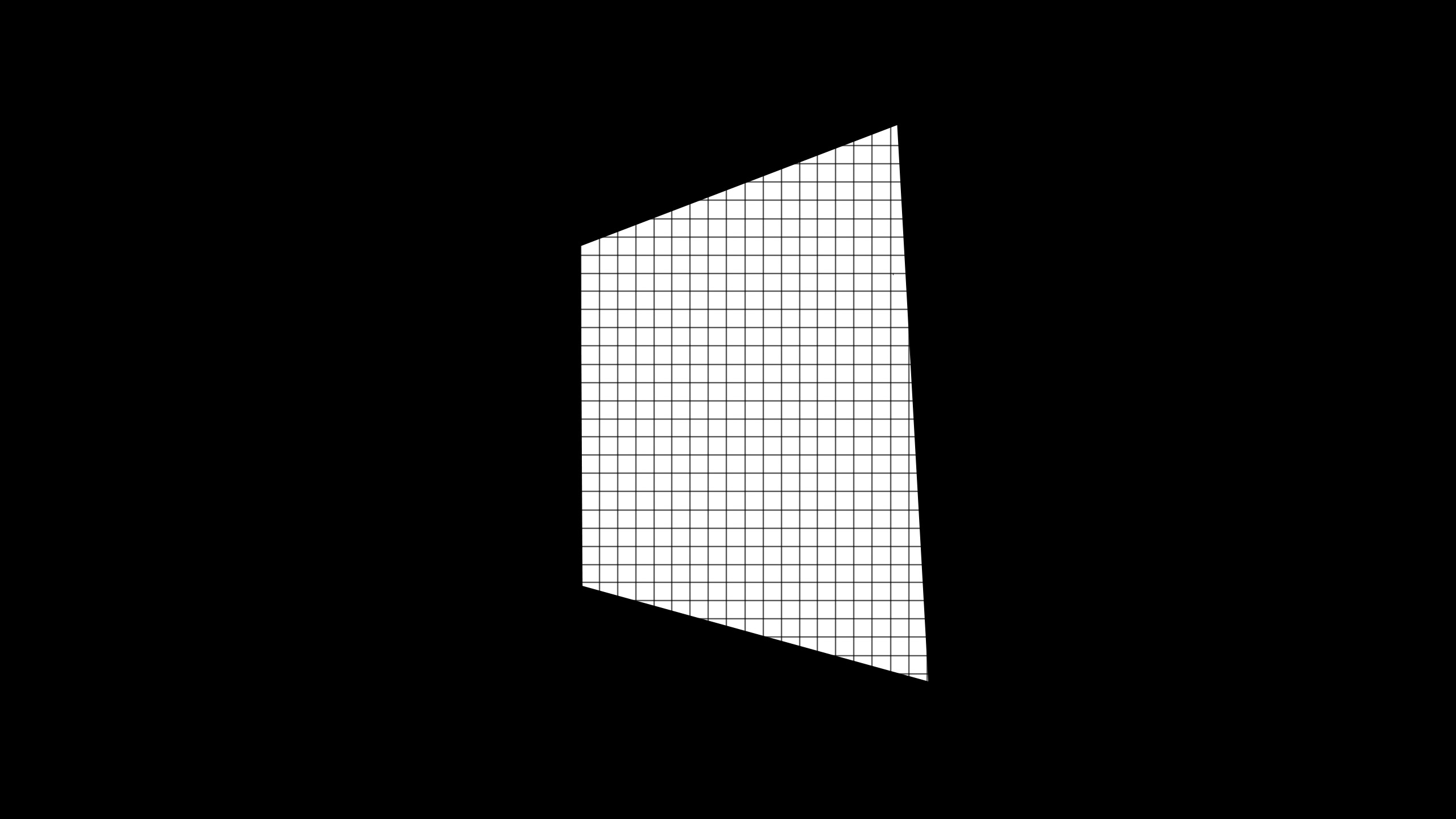Here's where some of the magic happens:

the system fills the space connecting these dots
and calculates what pixels on your display
need to be drawn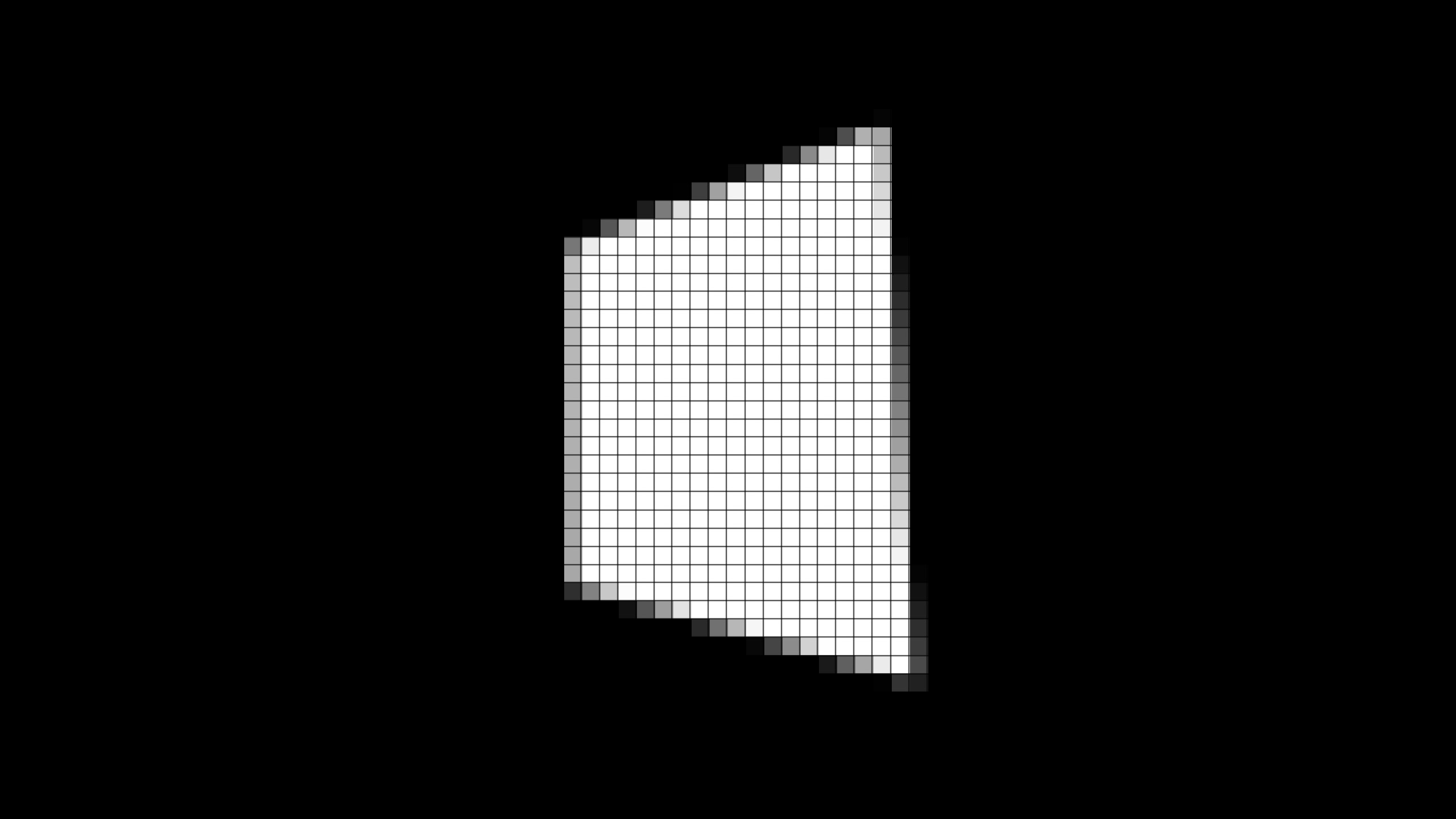The rest is up to the fragment shader

It gets called for each of these small squares,
and the correct output color is calculated.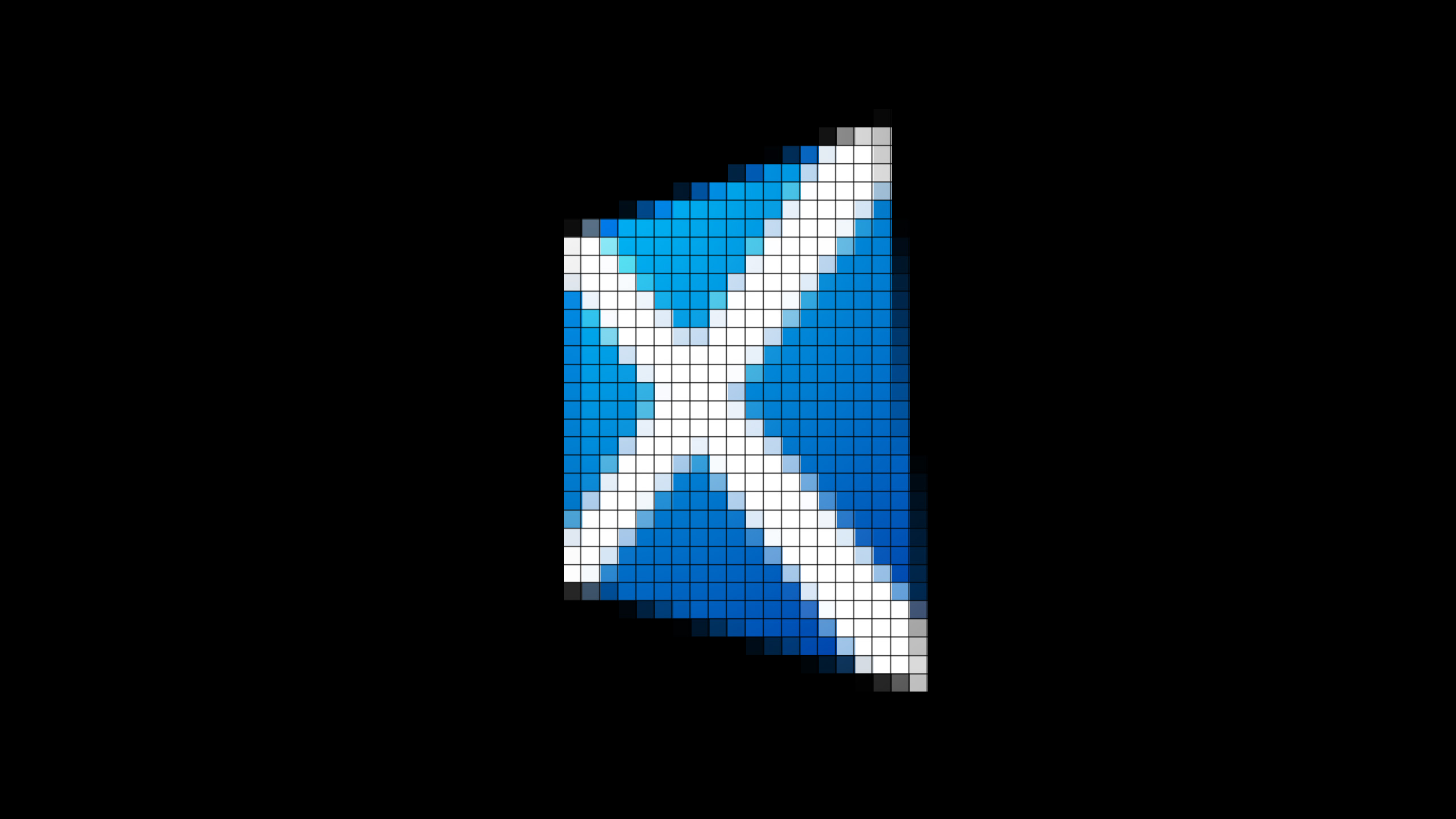The result is a framebuffer with an image!

So we have several operations
that can be done per vertex,
and some that can be done per fragment

"per vertex" is usually less expensive than "per fragment"
(several thousand vertices vs several million pixels)

# GPU

the Graphics Processing Unit take this idea
and makes it faster

Data is defined in specific formats, and transfered
from main CPU memory to GPU memory

Vertices, faces, matrices, textures...

Groups of geometries are bound with programs
and related data like light color, texture, etc.

You can think of it as a material:
tells the system how to represent a geometry

Geometry + material = shaded object

The main program issues commands to the GPU
to draw each object

"Your mission, should you choose to accept it
it's specifying those commands"

GPUs have several units that can execute
shader code and access data in the GPU memory

All those units run in parallel,
computing a vertex or a fragment,
much faster than other kinds of CPUs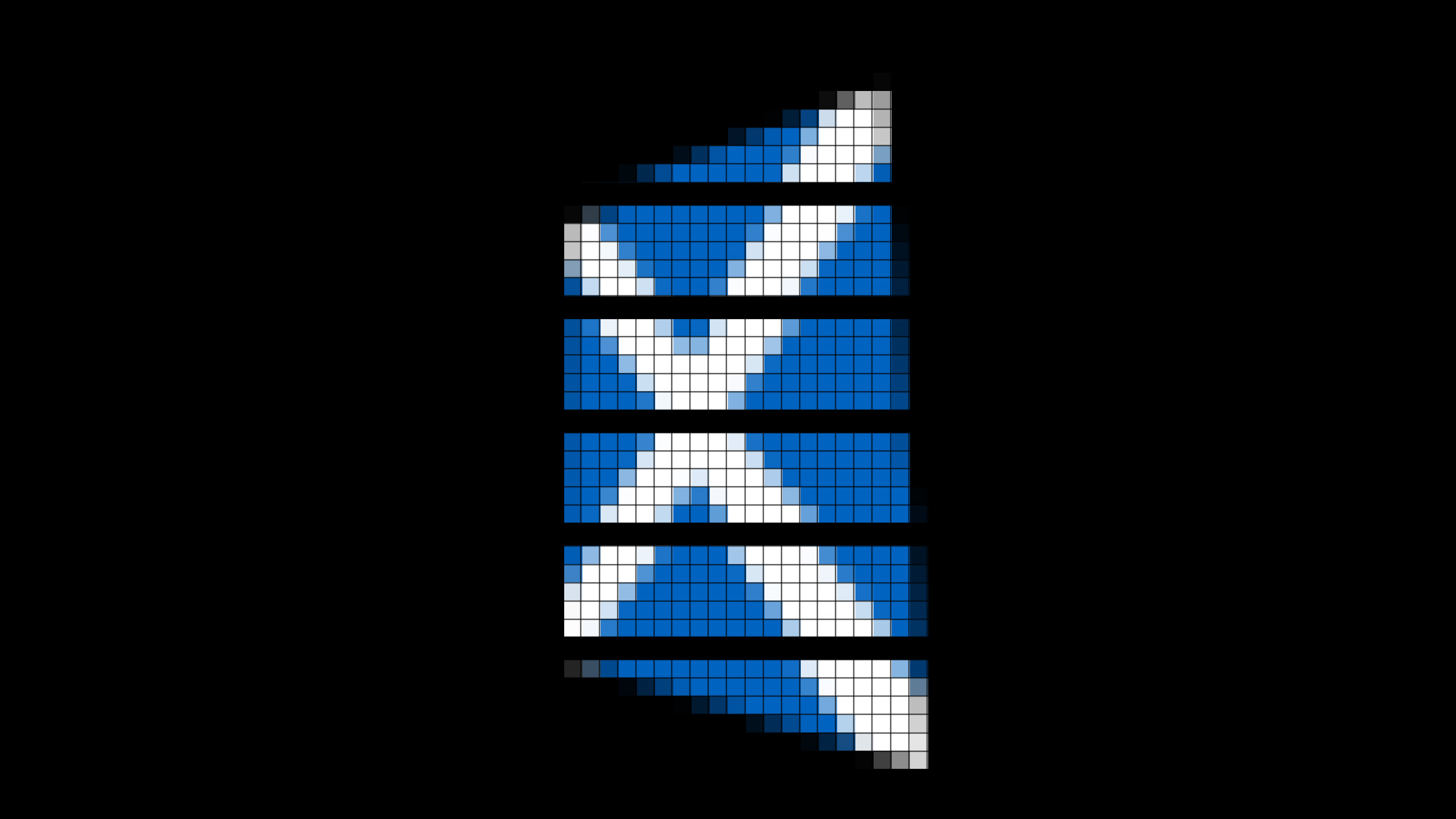Based on OpenGL ES 2.0

It's a JavaScript API

A program has a vertex and a fragment shader

It's easy! But it's even easier with three.js

# Standard material with three.js

``````
var scene = new THREE.Scene();
var aspectRatio = window.innerWidth / window.innerHeight;
var camera = new THREE.PerspectiveCamera( 75, aspectRatio, 0.1, 1000 );
camera.position.z = 5;

var renderer = new THREE.WebGLRenderer();
renderer.setSize( window.innerWidth, window.innerHeight );
document.body.appendChild( renderer.domElement );

var geometry = new THREE.BoxGeometry( 1, 1, 1 );
var material = new THREE.MeshBasicMaterial( {
});
var cube = new THREE.Mesh( geometry, material );

renderer.render( scene, camera );``````

# Three.js materials

## Some of them, at least

THREE.MeshBasicMaterial
THREE.MeshDepthMaterial
THREE.MeshLambertMaterial
THREE.MeshNormalMaterial
THREE.MeshPhongMaterial

# Custom material with three.js

``````
var scene = new THREE.Scene();
var aspectRatio = window.innerWidth / window.innerHeight;
var camera = new THREE.PerspectiveCamera( 75, aspectRatio, 0.1, 1000 );
camera.position.z = 5;

var renderer = new THREE.WebGLRenderer();
renderer.setSize( window.innerWidth, window.innerHeight );
document.body.appendChild( renderer.domElement );

var geometry = new THREE.BoxGeometry( 1, 1, 1 );
var material = new THREE.ShaderMaterial( {
uniforms: {
map: { type: 't', value: THREE.ImageUtils.loadTexture( 'map.png' ) }
},
} );
var cube = new THREE.Mesh( geometry, material );

renderer.render( scene, camera );``````

# Normal material | Vertex shader

``````
uniform mat4 modelViewMatrix;
uniform mat4 projectionMatrix;
uniform mat4 normalMatrix;

attribute vec3 position;
attribute vec2 uv;

varying vec2 vUv;

void main() {

vUv = uv;
gl_Position = projectionMatrix * modelViewMatrix * vec4( position, 1.0 );

}``````

# Normal material | Fragment shader

``````
uniform sampler2D map;

varying vec2 vUv;

void main() {

gl_FragColor = texture2D( map, vUv );

}``````

# Shader source in inline scripts

``````<script id="vertexShader" type="x-shader/x-vertex">
</script>

</script>

var material = new THREE.ShaderMaterial( {
} );
</script>
``````

## Postprocessing

It's a subcase of this technique

Create a quad that covers the screen,
set the current renderbuffer as source,
play with the values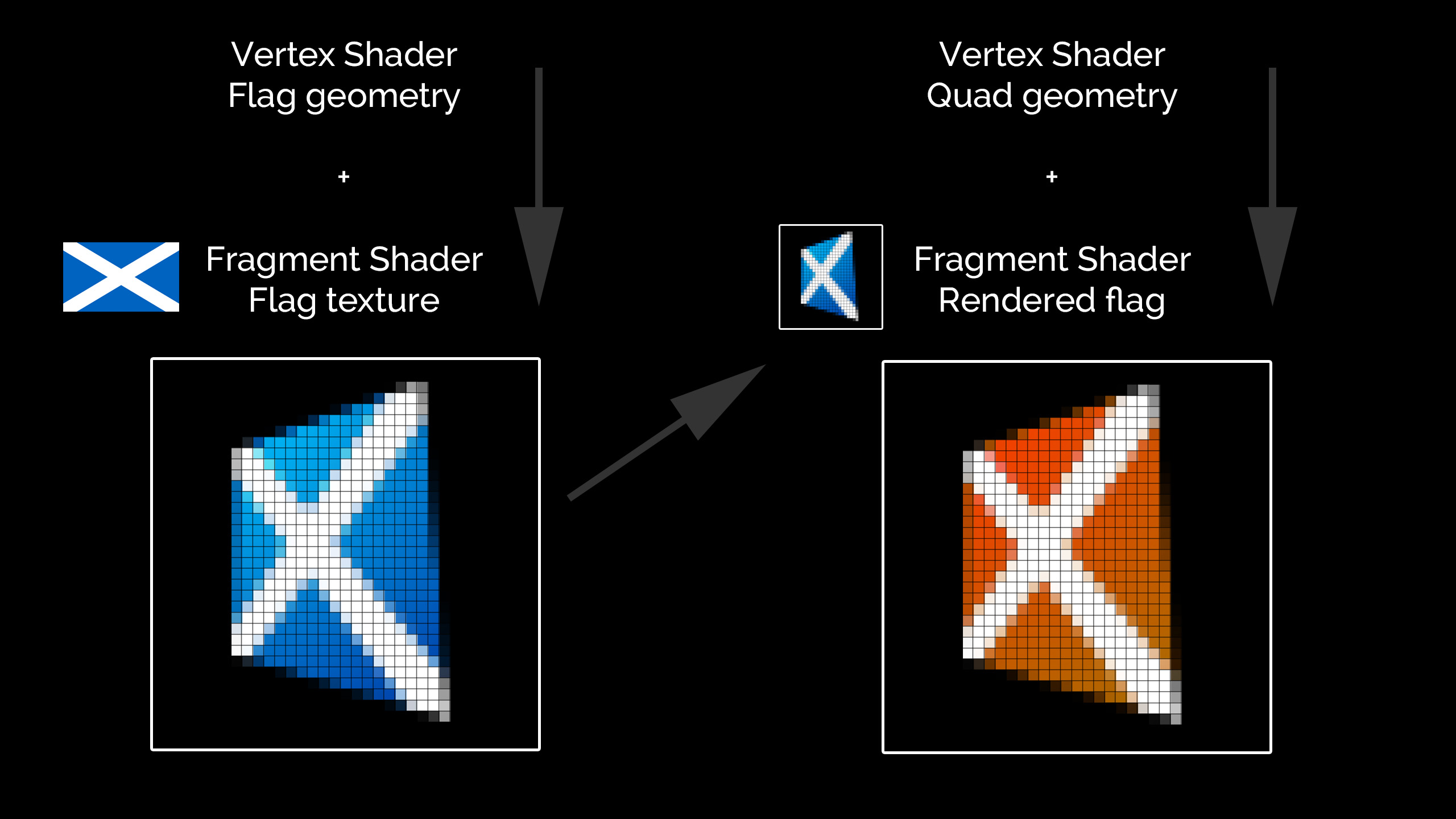Voilà, postprocessing!

GLSL Sandbox

GLSLBin

Shdr

# But we're not done yet!

We're not limited to graphical output!

We can use shaders to process arbitrary data

That's what we call GPGPU

General-purpose computing on graphics processing units

Frameworks and platforms

OpenCL (Open Computing Language),
CUDA (Compute Unified Device Architecture)

In the meantime,
we can do it with WebGL

It's a bit like postprocessing

First, our geometry is going to be a quad.
There's not going to be any perspective transformation

We want the number of output pixels to be exactly
the number of our input values.

We load our input data in textures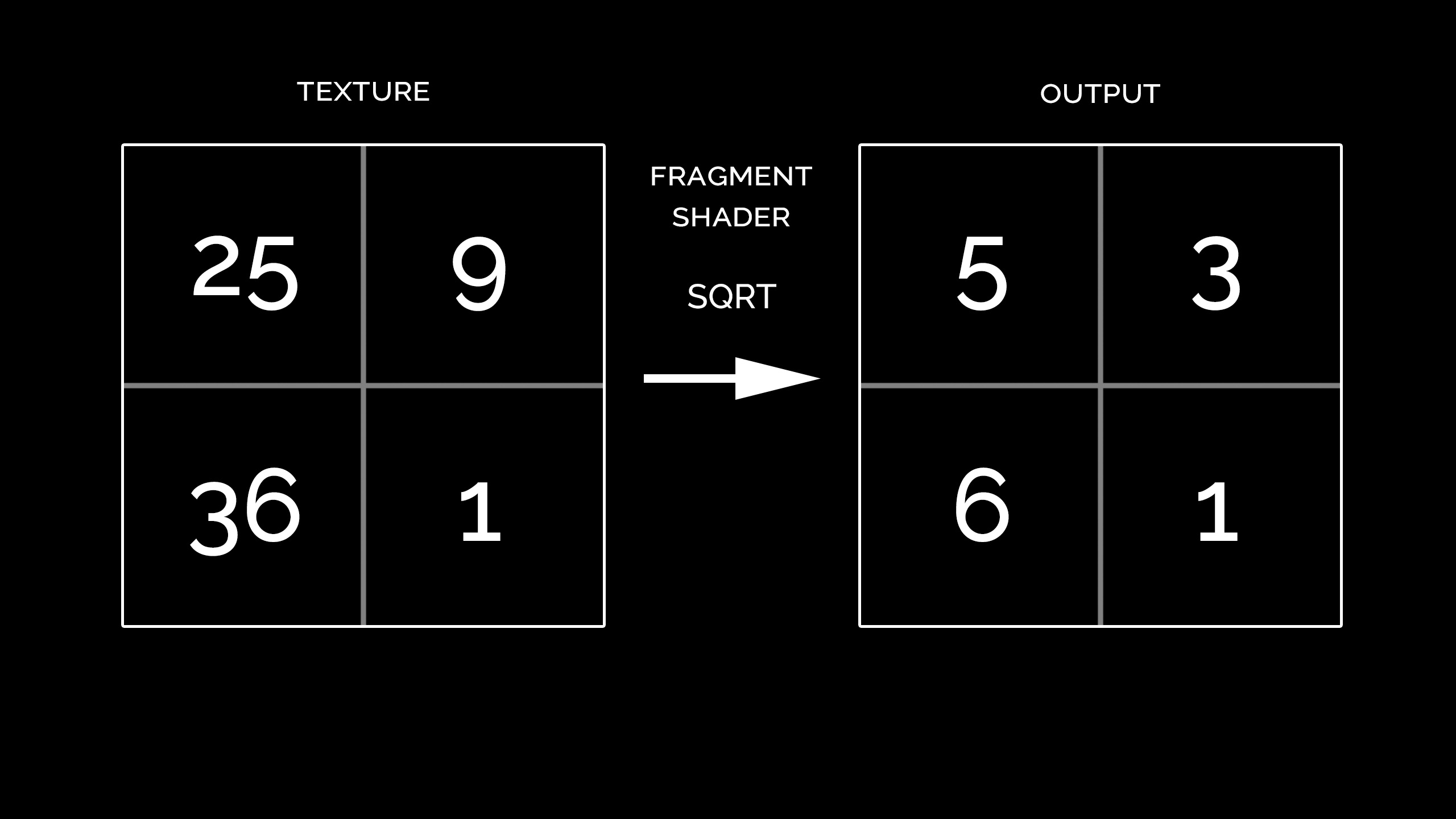Encoding data in textures is tricky

Images are usually vec4 specifying RGBA

Sometimes we can use float textures
but we can only read from 4-bytes buffer.

We have to encode numbers in 32 or 64 bits

Integers

 1 byte 8 bits 2^8 = 256 values unsigned: 0 to 255signed: -128 to 127 4 bytes 32 bits 2^32 = 4,294,967,296 values unsigned: 0 to 4,294,967,295signed: −2,147,483,648 to 2,147,483,647

None of these can hold decimal values

Floating point
IEEE Standard for Floating-Point Arithmetic (IEEE 754)

 single precision 32 bits ±1.18×10−38 to ±3.4×10^38 approx. 7 decimal digits double precision 64 bits ±2.23×10^−308 to ±1.80×10308 approx. 15 decimal digits

IEEE 754 (continued)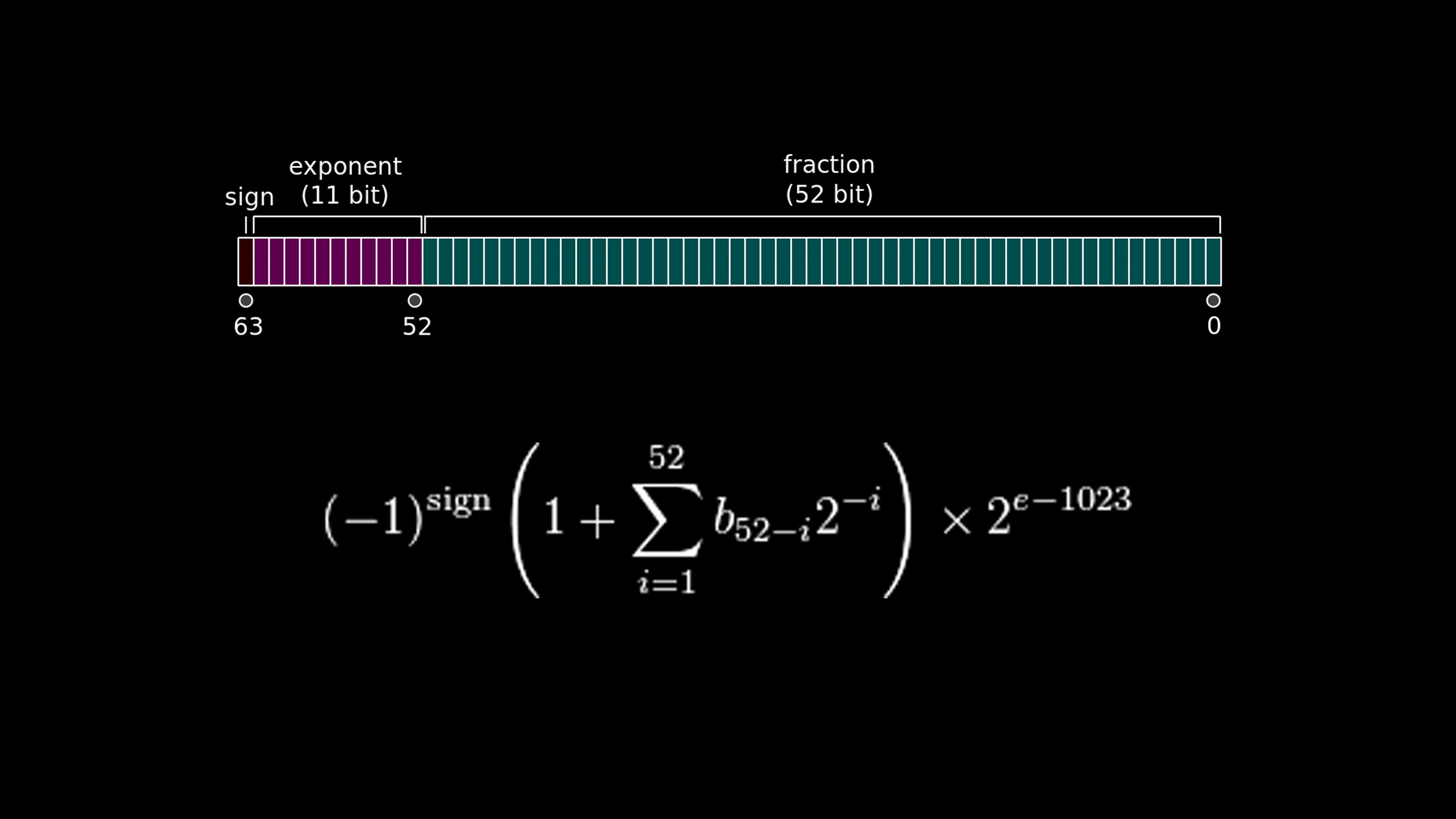anyhow

Preparing data

``````
var data = new Float32Array( width * height );
var inputData = new Uint8Array( data.buffer );

inputData,
width,
height,
THREE.RGBAFormat,
THREE.UnsignedByteType
);
``````

The vertex shader doesn't do much:

it makes sure the output is formatted as we expect,
an unprojected quad with the right size

``````
uniform mat4 modelViewMatrix;
uniform mat4 projectionMatrix;

attribute vec3 position;
attribute vec2 uv;

varying vec2 vUv;

void main() {

vUv = uv;
gl_Position = projectionMatrix * modelViewMatrix * vec4( position, 1.0 );

}``````

The fragment shader does the calculations

Reads from the input texture(s), pixel by pixel,
calculates in parallel and writes to output

``````
uniform sampler2D tInput;

varying vec2 vUv;

float calculate( float v ) {

return sqrt( v );

}

void main() {

vec4 val = decode_float( texture2D( tInput, vUv ) );
float res = calculate( val );
gl_FragColor = encode_float( res );

}``````

Running

``````
var renderer = new THREE.WebGLRenderer( { antialias: false } );
renderer.setSize( width, height );
renderer.setPixelRatio( 1 );

var scene = new THREE.Scene();
var camera = new THREE.OrthographicCamera(
width / - 2, width / 2,
height / 2, height / - 2,
-10000, 10000
);

var geometry = new THREE.PlaneBufferGeometry( width, height );

var result = createRenderTarget( width, height );

renderer.render( scene, camera, result );``````

Rendered data is in GPU memory

``````
var pixels = new Uint8Array( 4 * width * height );
var gl = renderer.context;
var framebuffer = result.__webglFramebuffer;
gl.bindFramebuffer( gl.FRAMEBUFFER, framebuffer );
gl.viewport( 0, 0, width, height );
gl.readPixels( 0, 0, width, height, gl.RGBA, gl.UNSIGNED_BYTE, pixels );
gl.bindFramebuffer( gl.FRAMEBUFFER, null );

var output = new Float32Array( pixels.buffer );``````

array.map

finding patterns

sound generation

# Jaume Sanchez

Technical Director / Creative Technologist @ B-REEL

clicktorelease.com

Questions?